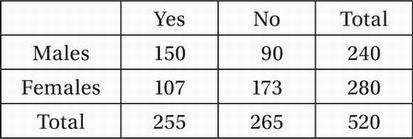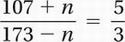# SAT Math Multiple Choice Question 779: Answer and Explanation

### Test Information

Question: 779

14.The table above shows the results of a survey of 520 adults who were asked whether they approved of a recent state budget proposal.

If n of the females had voted yes instead of no, the ratio of yes votes to no votes would have been exactly the same for the females as for the males. What is the value of n?

• A. 62
• B. 66
• C. 68
• D. 70

Explanation:

C

Problem Solving/Data Analysis (tables/ratios) MEDIUM-HARD

For the males, the ratio of yes votes to no votes is 150:90 = 5:3. If n of the females had shifted their votes from no to yes, then 173 - n would have voted no and 107 + n would have voted yes. If this ratio is then equal to the ratio for males,Cross-multiply:

321 + 3n = 865 - 5n